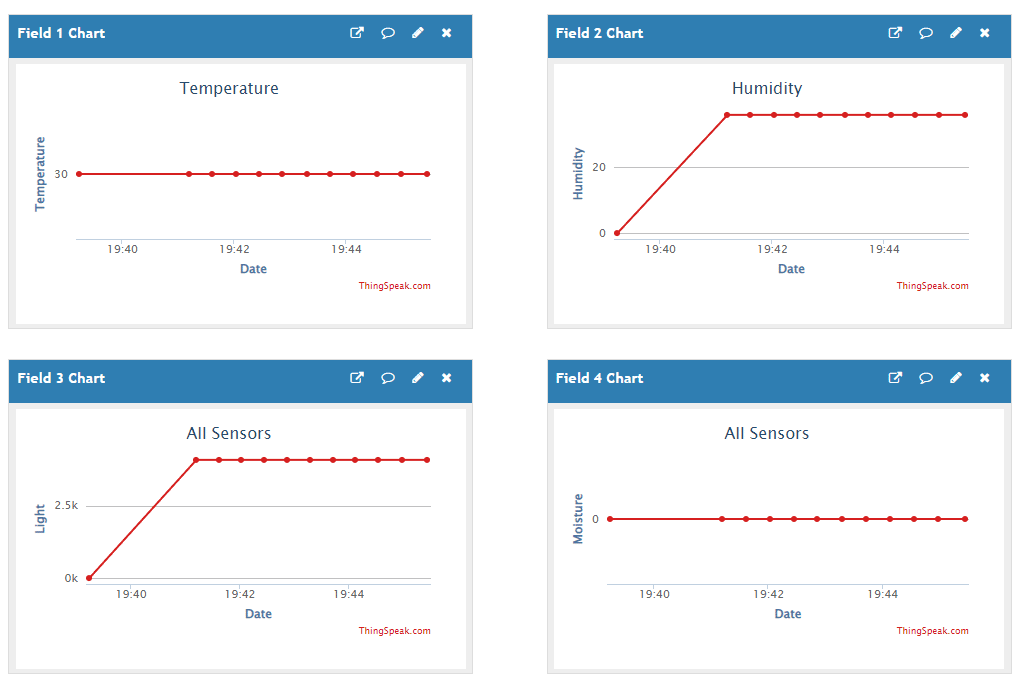# Create an IoT House Monitoring System with ThingSpeak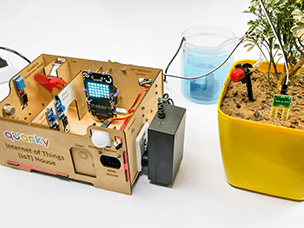Example Description
This tutorial will show you how to create an IoT House Monitoring system that sends humidity, temperature, plant soil moisture, gas, and light sensor data to the cloud on a ThingSpeak channel.

This example shows how to create an IoT House Monitoring system that sends humidity, temperature, plant soil moisture, gas, and light sensor data to the cloud on the ThingSpeak channel.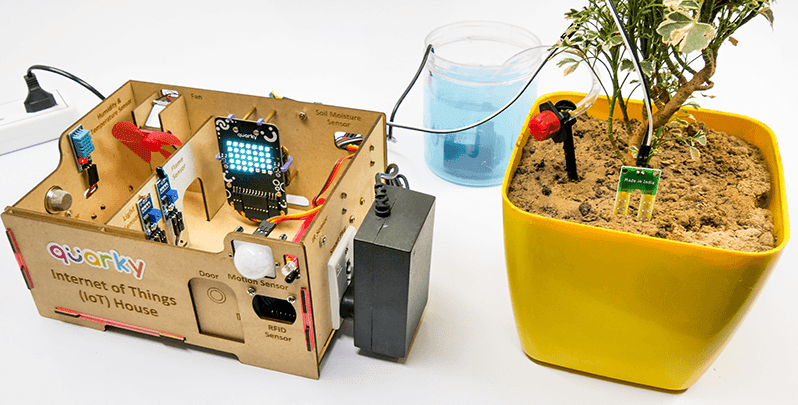#### ThingSpeak Channel

Create a channel on ThingSpeak with 5 fields – Temperature, Humidity, Light, Gas, and Moisture.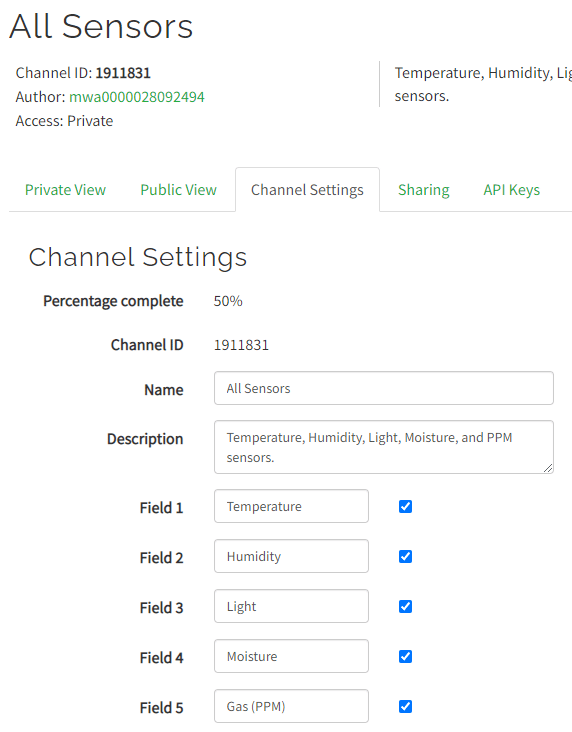#### Sensor Connection to Quarky

We are using 4 sensors in this project:

1. DHT sensors have 3 pins: GND, VCC, and Signal. You have to connect the following 3 pins to the Quarky Expansion Board:
1. GND to Ground Pin of Quarky Expansion Board
2. VCC to 3.3V or VCC Pin of Quarky Expansion Board
3. Signal to the D3 (Digital Pin) of the Quarky Expansion Board
2. LDR sensors have 4 pins: GND, VCC, DO, and AO. You have to connect the following 3 pins to the Quarky Expansion Board:
1. GND to Ground Pin of Quarky Expansion Board
2. VCC to 3.3V or VCC Pin of Quarky Expansion Board
3. AO to the A3 (Analog Pin) of the Quarky Expansion Board
3. The gas sensor has the following connections:
1. GND Pin connected to GND of the Quarky Expansion Board.
2. VCC Pin connected to VCC of the Quarky Expansion Board.
3. AO (Signal Pin) connected to Analog Pin A1 of the Quarky Expansion Board
4. Moisture Sensor: The moisture sensor provides real-time moisture reading from the soil. The moisture sensor connections are as follows:
1. GND Pin connected to GND of the Quarky Expansion Board.
2. VCC Pin connected to VCC of the Quarky Expansion Board.
3. AO (Signal Pin) connected to Analog Pin A2 of the Quarky Expansion Board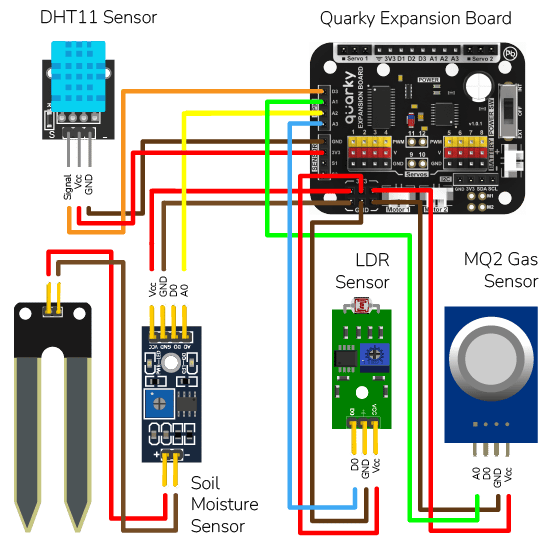#### Python Code

This code connects to an IoT house with sensors, reads the sensor values, prints them, and sends them to data storage:

1. It first imports the necessary packages and creates the objects required to connect to the cloud.
2. It then establishes a connection to the ThingSpeak cloud using a given channel number, read key and write key.
3. Variables used by the code are initialized and two user-defined functions are defined. One is used to calibrate the gas sensor and the other is used to read the gas sensor.
4. The code then sets the pin to read the moisture sensor and starts a loop that reads the sensors, and sends them to the data storage.
5. Finally, it waits for 20 seconds before sending the next set of data.
``````# The following code connects to a device, reads sensor values and sends them to a data storage.
# First, the necessary packages are imported.
import time
import math

# Then, three objects are created: one for the IoT house with the sensors and one for the ThinkSpeak and one for Quarky.
house = IoTHouse()
ts = ThingSpeak()
quarky = Quarky()

# Next, the code connects to the ThingSpeak cloud using the given channel number, read key and write key.
ts.connecttoThingSpeak(1911831, "P8YVTC1UGYJZIIZY", "UP0XIQDW8FZA8I0G")

# Variables used by the code are created and initialized.
b = 1.413
m = -0.473

# User Defined Functions

Sensor_Value = 0.1
# This loop reads the sensor value 20 times.
for i in range(0, 20):
Sensor_Value += house.gassensorvalue("A1")
# This line calculates the average of the 20 readings.
Sensor_Value = (Sensor_Value / 20)

# This line calculates the RS_of_Air variable.
RS_of_Air = (4095 - Sensor_Value) / Sensor_Value

# This line calculates the R0 variable.
R0 = RS_of_Air / 9.8
time.sleep(1)

# This function reads the gas sensor.
# This line reads the sensor value.
Sensor_Value = house.gassensorvalue("A1")
# This line checks if the sensor value is 0.
if (Sensor_Value == 0):
# This line returns 0 if the sensor value is 0.
return 0
else:
# This line calculates the RS_of_Air variable.
RS_of_Air = ((4095 - Sensor_Value) / Sensor_Value)
# This line calculates the RS_RO_Ratio variable.
RS_RO_Ratio = (RS_of_Air / R0)
# This line calculates the PPM_in_Log variable.
PPM_in_Log = (((math.log(RS_RO_Ratio)) - b) / m)
# This line calculates the PPM variable.
PPM = (pow(10, PPM_in_Log))
# This line returns the PPM value.
return PPM

# This line sets the pin to read the moisture sensor.
house.setmoisturepin("A3")

# This loop reads the sensors, prints their values and sends them to the data storage.
while True:
# This line reads the temperature from the DHT sensor on pin D3 and sends it to the data storage.
Temperature = house.dhtmeasure(1, "D3")

# This line reads the humidity from the DHT sensor on pin D3.
Humidity = house.dhtmeasure(2, "D3")

# This line reads the light from the light sensor on pin A2.
Light = house.ldrvalue("A2")

# This line reads the moisture from the moisture sensor.

# This line calls the Get_Gas_Sensor_Reading() function to read the gas sensor.